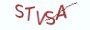Java基础教程

# 10.2.1 数组的length属性

Java数组的常用属性主要有length,表示数组的长度。数组的length属性保存了数组的元素数目。如果是一维数组，那么很好理解，数组中有多少个元素，length的值就为多少。例如下表所示的一维数组：

 1 2 3 4 5 6 7 8 9

# 10.2.2 数组的索引访问

`dataType element = array[index];`

```public class HelloJava{
public  static void main(String[] args) {
// 定义一个长度为5的一维数组
int[] numbers = new int;
// 将索引0对应的元素设置为1
numbers = 1;
// 将索引1对应的元素设置为2
numbers = 2;

// 访问索引0对应的元素值
System.out.println(numbers);
}
}```

# 10.2.3 在循环中遍历数组

```public class HelloJava{
public  static void main(String[] args) {
// 定义一个长度为5的一维数组
int[] numbers = new int;
for(int index=0; index < numbers.length; ++index) {
// 将index位置的元素值设置为index
numbers[index] = index;
}
}
}```

```public class HelloJava{
public  static void main(String[] args) {
// 定义整型数组numbers
int[] numbers = {1, 2, 3, 4, 5};
for(int index=0; index < numbers.length; ++index) {
// print方法在输出的时候不会换行
System.out.print(numbers[index]);
System.out.print(' ');
}
}
}```

```public class HelloJava{
public  static void main(String[] args) {
// 定义二维整型数组numbers
int[][] numbers = {{1, 2, 3}, {4, 5, 6, 7}, {8, 9, 10}};
// 通过两层for循环来遍历二维数组中的所有元素
for(int outer_index = 0; outer_index < numbers.length; ++outer_index){

// 二维数组中的每一个元素是一个一维数组，再通过一维数组的length来得到元素的数目
for (int inner_index = 0; inner_index < numbers[outer_index].length; ++inner_index){
System.out.print(numbers[outer_index][inner_index]);
System.out.print(' ');
}
// 执行println方法来换行
System.out.println();
}
}
}```

# 10.2.4 foreach循环结构

```for (dataType element : array) {
;
}```

dataType表示元素的数据类型，element表示元素，array表示数组。

```public class HelloJava{
public  static void main(String[] args) {
// 定义整型数组numbers
int[] numbers = {1, 2, 3, 4, 5};
for(int element: numbers){
System.out.print(element);
System.out.print(' ');
}
}
}```

```public class HelloJava{
public  static void main(String[] args) {
// 定义二维整型数组numbers
int[][] numbers = {{1,2, 3}, {4, 5, 6}, {7, 8, 9}};
for (int [] numbers_: numbers) {
for (int element: numbers_) {
System.out.print(element);
System.out.print(' ');
}
System.out.println();
}
}```

# 10.2.5 内置的Arrays类

Java提供了一个Arrays类，该类位于java.utils包中，用来高效、快捷的处理数组。下表所示为Arrays类的常用方法：

 方法名 描述 Arrays.toString(Object[] array) 将数组array中的元素拼接为字符串并返回 Arrays.fill(Object[] array, Object val) 将数组array全部填充为value值 Arrays.sort(Object[] array) 对数组array进行排序 Arrays.binarySearch(Object[] array, Object key) 使用二分查找算法在数组array中查找key值 Arrays.equals(Object[] array1, Object[] array2) 判断数组array1与array2是否相等，相等返回true,否则返回false。两数组只有在元素都相同，且排列顺序一致的情况下才相等。 Arrays.copyOf(Object[] array, int newLength) 构建一个新数组，并复制array中的前count个元素。 Arrays.copyOfRange(Object[] array, int start, int end) 构建一个新数组，并复制array中的[start, end)范围中的元素。

```// 需先通过import指令来导入Arrays类
import java.util.Arrays;

public class HelloJava{
public  static void main(String[] args) {
// 定义一个长度为5的一维数组
int[] numbers = new int;
// 通过fill方法将数组的所有元素填充为5
Arrays.fill(numbers, 5);

// 通过toString方法将数组转换为字符串，方便输出
System.out.println(Arrays.toString(numbers));
}
}```

```// 需先通过import指令来导入Arrays类
import java.util.Arrays;

public class HelloJava{
public  static void main(String[] args) {
// 定义一个长度为7的一维数组
int[] numbers = {5, 2, 1, 4, 7, 9, 11};
// 通过copyOf方法来复制数组
int[] newNumbers = Arrays.copyOf(numbers, 5);

// 通过sort方法来排序
Arrays.sort(newNumbers);
// 通过toString方法将数组转换为字符串，方便输出
System.out.println(Arrays.toString(newNumbers));
}```

# 10.2.6 课后习题

(1) 定义一个一维的整型数组，并将其初始化为奇数的集合

(2) 分别使用for循环和foreach循环来遍历(1)题中的数组

(3) 查找与Java随机数处理相关的资料，然后定义一个二维的整型数组，该数组中的所有元素设为随机值。

(4) 对(3)题中的二维数组进行排序，要求二维数组中的每个元素都是升序排序。

# 10.2.7 高薪就业班

`(1) Python后端工程师高薪就业班，月薪10K-15K，免费领取课程大纲(2) Python爬虫工程师高薪就业班，年薪十五万，免费领取课程大纲(3) Java后端开发工程师高薪就业班，月薪10K-20K, 免费领取课程大纲(4) Python大数据工程师就业班，月薪12K-25K,免费领取课程大纲`## 注册账号